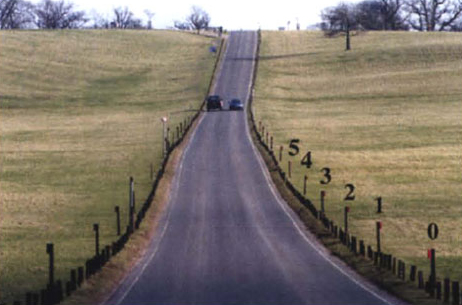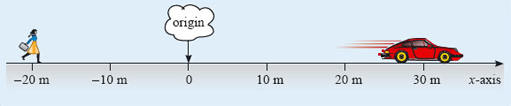Describing motion along a line

Start this free course now. Just create an account and sign in. Enrol and complete the course for a free statement of participation or digital badge if available.

Free course

# 2.2 Describing positions along a line

To take a definite case, consider a car moving along a straight horizontal road. The car can be modelled as a particle by supposing the particle to be located at, say, the midpoint of the car. It is clearly convenient to measure the progress of the car with respect to the road, and for this purpose you might use the set of uniformly spaced red-topped posts along the right-hand side of the road (see Figure 5). The posts provide a way of assigning a unique position coordinate to the car (regarded as a particle) at any instant. The instantaneous position coordinate of the car is simply the number of the nearest post at that moment.Figure 5: A long straight road and a set of uniformly spaced posts along it

When used in this way the posts provide the basis of a one-dimensional coordinate system - a systematic means of assigning position coordinates along a line. Taken together, the posts constitute an axis of the system; a straight line along which distances can be measured. One point on the axis must be chosen as the origin and assigned the value 0. Points on one side of the origin can then be labelled by their distance from the origin (10 m or 20 m say), while points on the other side are labelled by minus their distance from the origin (−10 m or −20 m for example). Conventionally, we might represent any of these values by the algebraic symbol x, in which case it would be called the x-coordinate of the corresponding point on the axis, and the axis itself would be called the x-axis.

Figure 6 shows an example, corresponding to a particular choice of origin, and with x increasing smoothly from left to right, as indicated by the arrow. Note that the numbers x increase from left to right everywhere on the x-axis, not only on the positive segment. Thus for example −﻿10 is larger than −﻿20, and so if you subtract −﻿20 from −﻿10 you get −﻿10 − (﻿−﻿20﻿) = 10, a positive number. At the time illustrated the car is at x = 30 m, and a pedestrian (also modelled as a particle) is at x = −20 m. Notice that it is essential to include the units in the specification of x. It makes no sense to refer to the position of the car as being '30', with no mention of the units, i.e. metres. In one dimension, the specification of a physical position x consists of a positive or negative number multiplied by an appropriate unit, in this case the metre.Figure 6: A choice of x-axis. Setting up the axis involves choosing an origin and a direction of increasing x (from left to right). Distances are measured in metres (m)

It is worth emphasising that setting up an x-axis involves some degree of choice. The origin and the direction of increasing x are both chosen in an arbitrary way, usually so as to simplify the problem. The choice of units of measurement is also arbitrary, though usually guided by convention. Even the decision to call the axis an x-axis is arbitrary; x is conventional, but not compulsory. It does not really matter what choices are made, but it is essential to stick to the same choices throughout the description of a given motion.

The conventions about units deserve special attention, so they have been set apart from the main text in Box 1.

## Box 1 Introducing SI units

The standard way for scientists to measure lengths, distances and positions is in metric units (metres, millimetres, kilometres, etc., as opposed to miles, feet or inches). This is part of an internationally agreed system of units known as SI (which stands for the Système Internationale). This course uses SI units throughout.

The standard SI abbreviation for the metre is m. Since 1983 the speed of light travelling in a vacuum has been defined to be exactly

2.99792458 × 108 metres per second.

This is an internationally agreed definition, not the result of a measurement, so the metre may be similarly defined as the distance that light travels through a vacuum in (1 / 299 792 458) second. Typical lengths of interest to physicists range from the diameter of the atomic nucleus, which is about 10−15 m, to the diameter of the visible Universe, which is about 1027 m.

In view of the wide range of lengths that are of interest, it would be inconvenient to use only the metre for their measurement. To avoid this there are standard SI multiples and SI submultiples that may also be used. You will already be familiar with some of these; the prefix kilo means 103 as in kilometre and the prefix milli stands for 10−3 as in millimetre. Table 1 gives the standard SI multiples; you are not expected to remember all of them but it is certainly worth learning the more common ones.

Another example of an SI unit is the second which is the unit of time. The standard abbreviation for the second is s. (Notice that, like m, this symbol is always lower case. The distinction does matter.) The time values of interest to experimental physicists range from the 10−24 s duration of certain events in subatomic physics, to the present age of the Universe, which is about 1018 s, though speculations about the birth and death of the Universe have involved times ranging from 10−45 s to 10140 s, or more.

### Table 1: Standard SI multiples and submultiples

MultiplePrefixSymbol for prefixSubmultiplePrefixSymbol for prefix
1012teraT10−3millim
109gigaG10−6microμ
106megaM10−9nanon
103kilok10−12picop
10010−15femtof

Having chosen a convenient x-axis and selected units of length and time, we are well placed to describe the position of a particle as it moves along a straight line. All we need to do is to equip ourselves with a clock, choose an origin of time (that is, an instant at which the time t = 0 s) and note the position of the particle at a series of closely spaced intervals. Table 2 shows typical results for the kind of car in Figure 6. The use of an oblique slash (/) or solidus in the column headings in Table 2 is another convention. It reminds us that the quantity to the left of the solidus is being measured in the units listed on the right of the solidus.

## Table 2: The position coordinate x of the car in Figure 6 at various times t

t/sx/m
00
51.7
106.8
1515
2026
2539
3053
3568
4084
4599
50115
55131
60146

In fact, if you remember that a physical quantity such as x represents the product of a number and a unit of measurement, as in 20 m, for example, you can see that x/m may be thought of as indicating 'the value of x divided by 1 metre', which would just be the number 20 if x was 20 m. It therefore makes good sense to see that the entries in the x/m column of Table 2 are indeed just numbers. In effect, x/m indicates that the units have been divided out. Take care always to remember that symbols such as x, that are used to represent physical quantities, conventionally include the relevant units while quantities such as x/m are purely numerical.

S207_2